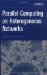# 7.4. UNDERLYING MODELS AND THE MAPPING ALGORITHM

## 7.3. ALGORITHMIC PATTERNS

The performance model of the parallel algorithm presented in Section 7.1 takes into account the contribution of communication operations to the total execution time. This characteristic makes it much more realistic compared to the basic model presented in Chapter 6. The main parameters of this model are as follows:

• The number of parallel processes executing the algorithm.

• The absolute volume of computations performed by each process.

• The absolute volume of data transferred between each pair of processes during the execution of the algorithm.

However, one more important feature of the parallel algorithm that has a significant impact on its execution time is still not reflected in this model. That feature is the order of execution of computations and communications by the parallel processes of the algorithm.

The model says nothing about how exactly parallel processes are interacting during the execution of the algorithm. Therefore the mpC compiler must make some assumptions about the interaction in order to calculate the execution time of the algorithm. Namely, to calculate the execution time, the compiler assumes that

• each process executes all its computations in parallel with other processes,

• the processes execute all the communications in parallel, and

• a synchronization barrier exists between the execution of the computations and the communications.

This assumption is satisfactory only for a restricted class of parallel algorithms. In many parallel algorithms there are data dependencies among computations performed by different processes. One process may need data computed by other processes in order to start its computations, which serializes certain computations performed by different parallel processes. As a result the real execution time of the algorithm will be longer than the execution time calculated proceeding from the assumption about strictly parallel execution of computations.

On the other hand, some parallel algorithms can overlap computations and communications to achieve better performance. The real execution time of those algorithms will be shorter than the execution time proceeding from the assumption that computations and communications do not overlap.

Thus, when calculation of the execution time of different parallel algorithms is based on the same scenario of interactions of parallel processes during execution of the algorithm, the calculated execution time may be not accurate. This may lead to the mapping of the algorithms to the executing network of computers, which is far removed from optimal mapping. An obvious example is an algorithm with fully serialized computations performed by different processes. The optimal mapping should always assign all the processes to the fastest physical processor. Nevertheless, the assumption above could lead, as it often does, to mapping that involves all available processors.

The mpC language allows the programmer to specify the interactions of the parallel processes during execution of the parallel algorithm. This specification is a part of the network type’s definition. A new type of declaration, a scheme declaration, is introduced. It describes the interactions of abstract processors during the execution of the specified algorithm.

For example, the network type definition

` nettype Nbody(int m, int k, int n[m]) {    coord I=m;    node { I>=0: bench*((n[I]/k)*(n[I]/k));};    link { I>0: length*(n[I]*sizeof(Body)) [I]->;};    parent ;    scheme    {       int i;       par (i=0; i<m; i++) 100%%[i];       par (i=1; i<m; i++) 100%%[i]->;    }; };`

includes a scheme declaration. The definition describes a performance model of the parallel N-body simulation algorithm presented in Section 7.1. The scheme declaration just says to the mpC compiler that first all abstract processors perform in parallel 100 percent of computations that should be performed, and then all the processors, except the host-processor, send in parallel 100 percent of data that should be sent to the host-processor.

In this case the scheme declaration is redundant because the scenario it describes is identical to the default scenario used by the mpC compiler. The next network type definition describes the algorithm of parallel multiplication on a heterogeneous network of matrix A and the transposition of matrix B also presented in Section 7.1:

` nettype ParallelAxBT(int p, int n, int r, int d[p]) {    coord I=p;    node { I>=0: bench*((d[I]*n)/(r*r)); };    link (J=p) { I!=J: length*(d[I]*n*sizeof(double)) [J]->[I]; };    parent ;    scheme    {       int i, j, PivotProcessor=0, PivotRow=0;       for(i=0; i<n/r; i++, PivotRow+=r)       {          if(PivotRow>=d[PivotProcessor])          {             PivotProcessor++;             PivotRow=0;          }          for(j=0; j<p; j++)             if(j!=PivotProcessor)                (100.*r/d[PivotProcessor])%%[PivotProcessor]->[j];          par(j=0; j<p; j++)             (100.*r/n)%%[j];       }    }; };`

This definition of the network type ParallelAxBT also includes a scheme declaration. The scheme declaration simply says that

• the algorithm consists of n/r successive steps, and

• at each step a row of blocks (the pivot row) of matrix B, representing a column of blocks of matrix BT, is communicated (broadcast) vertically, and all abstract processors compute the corresponding column of blocks of matrix C in parallel.

Note that this algorithm was defined exactly the same way in Section 6.10 (see also Figure 6.2). The mpC compiler uses this information to estimate with higher accuracy the execution time of this algoirhtm. The rest of the code of the corresponding mpC program is entirely the same as it is given in Section 7.1.

The scheme declarations in the two examples above are relatively simple. They just reflect the relative simplicity of the underlying parallel algorithms. In general, the mpC language allows the programmer to describe quite sophisticated heterogeneous parallel algorithms by a wide use of parameters, locally declared variables, expressions, and statements.Parallel Computing on Heterogeneous Networks (Wiley Series on Parallel and Distributed Computing)
ISBN: B000W7ZQBO
EAN: N/A
Year: 2005
Pages: 95